# SSC CGL EXAMS 2018 | Reasoning Ability Practice Questions (Day-9)

Dear Aspirants, Here we have given the Important SSC Exam 2018 Practice Test Papers. Candidates those who are preparing for SSC 2018 can practice these questions to get more confidence to Crack SSC 2018 Examination.

[WpProQuiz 4219]

1) Rani correctly remembers that she took leave after 21st January and before 27th January. Her colleague Reena took leaves 23rd January but Rani was present on that day. It 24th January was a public holiday and 26th January was Sunday, then on which day ‘m’ January did Rani take leave?

a) 22nd January

b) 25th January

c) 22nd or 25th January

2) Find the missing character form the given alternatives.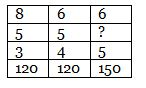a) 4

b) 5

c) 8

d) 10

3) At 6: 15, the hour hand and the minute hand of a clock form an angle of

a) 90°

b) 91.5°

c) 97.5°

d) 94.5°

4) P is mother of Q and Sister of R. R has a daughter S, Who is married T. V is the husband of P. How is V related to Q?

a) Uncle

b) Husband

c) Brother

d) Father

5) Mohan moved to his North – West side for 2 km. From there he turned 90° clockwise and moved 2 km. from there he turned 90° clockwise and travelled 2 km, than he would be in which direction from the original position?

a) South – East Region

b) North – East

c) South – East

d) Western Region

6) If 6#2 = 4, 2#6 = 4 and 14#12 = 13, Then find the  value of 4#24?

a) 8

b) 14

c) 20

d) 18

Direction ( 7 – 8): In the following question, a series is given, with one time missing. Choose the correct alternative from the given ones that will complete the series. ?

7) 97, 91, 79, 61, 37, ?

a) 7

b) 5

c) 4

d) 8

8) Z 9 A , X 7 D ,  T 3 J, R 1 M, ?

a) W6F

b) S3H

c) G9 V

d) V5G

9) In a certain code language ‘STAR’ is written as 6 \$ * 2 ‘ , ‘TORE’ is written as \$ 52 @ then how will ‘RATS’ be written in that language?

a) 3 * 5 \$

b) 3 * \$5

c) 3 \$ * 5

d) None of these

Directions (10 – 11): In the following questions, select the related word/number pairs from the given alternatives?

10) 7 : 13 : : 8 : ?

a) 9

b) 10

c) 11

d) 12

11) Adult: Baby : : Flower : ?

a) Seed

b) Bud

c) Fruit

d) Butter fly

12) In this series some letters are missing select the correct alternatives

a _ c b _ / _ b c_ c a b c b _

a) a c c b

b) b a a c a

c) b c a b c

d) b c b c c

13) Find the missing number from the given responses.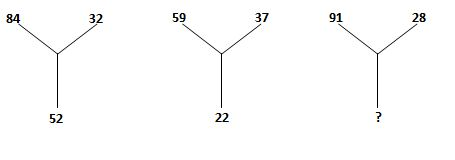a) 37

b) 73

c) 63

d) 87

14) In the question two statements are given, followed by conclusions, I and II. you have to consider the statements to be true even if it seems to be at variance from commonly known facts. You have to decide which of the given conclusions, if any, follows the given statement

Statement

All Milk is Acid

No milk is Tea

Conclusion:

Some Tea is Milk is a possibility

Some Acid is Milk is a possibility

a) Only Conclusion I follow

b) Only Conclusion II follow

c) Both Conclusion I and II follow

d) Neither Conclusion I nor II follow

15) Two year ago, Aadhya was three times as old as his son and two year hence, twice her age will be equal to five times that of her son. Find Aadhya’s present age.

a) 38 years

b) 36 years

c) 34 years

d) 42 years

Rani’s Leave day = 22, 23, 24, 25, 26

But Rani was present on 23rd. Also, it was holiday on 24th and 26th January was Sunday. Hence, possible data may be either 22nd or 25th January.

8 × 5 × 3 = 120

6× 5×4 = 120

6  × ? × 5 = 150

X = 150 / (6 * 5)

X = 5

Angle traced by hour hand in 12 h = 360°

Angle traced by hour hand in 25/4  h =

Angle traced by minute hand in 60 min = m= 360°

Angle traced by it in 15 m=

Required angle = 187.5 – 98 = 97.5°

Following family diagram can be down from the given information.

Now, it is clear that V is the Father of Q.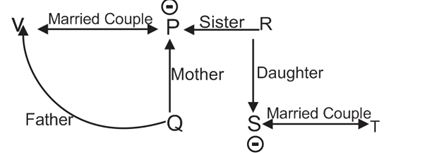Route followed by Ranju is given below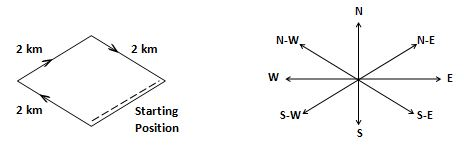Mohan will be in North – East direction from the original position.

6#2 = 4 = 6 + 2 = 8 / 2 = 4

2#6 = 4 = 2 + 6 = 8 / 2 = 4

14#12 = 13 = 14 + 12 = 26 / 2 = 13

4#24 = 4 + 24 = 28 / 2 = 14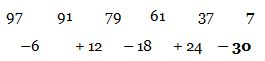Pattern of given alpha-numeric series b as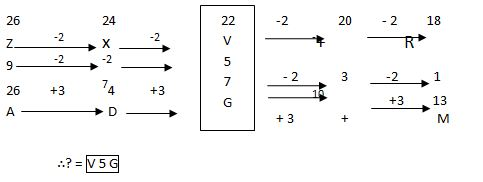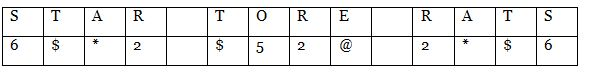72 = 49 => 4 + 9 =13

82 = 64 => 6 + 4 = 10

Former is the grown up state of letter.

a b c b c / a b c b c / abcbc

Difference of top most of both numbers will at bottom.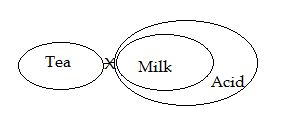According to question

3 (x – 2) = y – 2

3x – 6 = y – 2

3x – y = 6 – 2

3x – y = 6 – 2 = 4                  …….. (i)

After 2 years

5 (x + 2) = 2 (y + 2)

5x + 10 = 2y + 4

5x – 2y = 10 + 4                   …….. (ii)

From Equation (i) and (ii)

3x – y= 4 x 2 => 6x – 2y= 8 …….. (iii)

5x – 2y= – 6 x 1 = > 5x – 2y= – 6      …….. (iv)

Solving Eq. (iii) and (iv)

We get x = 14 and y = 38

### SSC Reasoning Ability Practice Questions – (Day-6)